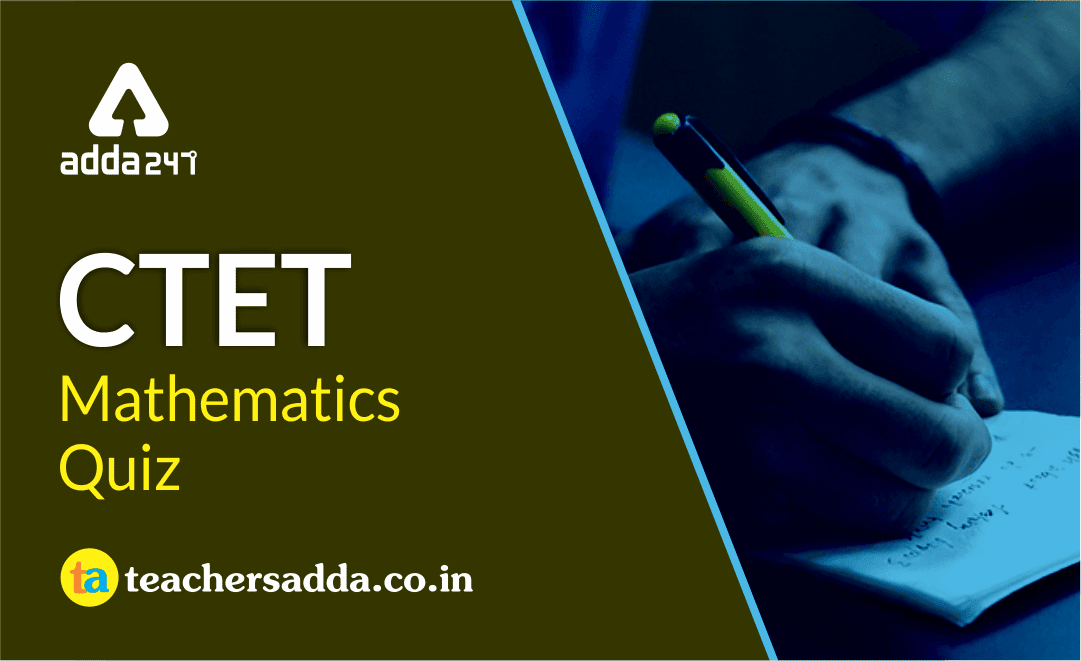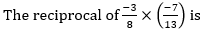Dear Students!!! There is most general as well as a scoring section in all the competitive entrance examinations in the teaching field i.e "Mathematics".Because in this section only one thing is work i.e your accuracy and that could be nourished with the daily practice. So, for this, we are providing you the daily quiz for all teaching exams i.e CTET Exam 2019DSSSB ,KVS,STET Exam.

Q1. The number of integers less than –3 but greater than –8 is
-2
-3
-4
6
Solution:
Required numbers, –4, –5, –6, –7

Q2. The distance between two places is 12 km. A map scale is 1 : 25000. The distance between the two places on the map, (in cm) is
24
36
48
60

Q3.104/21
–104/21
21/104
–21/104

Q4. The number of vertices in a polyhedron which has 30 edges and 12 faces is
12
15
20
24

Q5. When half of a number is increased by 15, the result is 39. The sum of digits of the original number is
6
7
9
12

Q6. In ∆PQT, PQ = PT. The points R and S are on QT such that PR = PS. If ∠PTS = 62° and ∠RPS = 34°, then measure of ∠QPR is
11°
13°
15°
17°

Q7. If for ∆ABC and ∆DEF the correspondence CAB ↔ EDF gives a congruence, then which of the following is not true?
AC = DE
AB = EF
∠A = ∠D
∠B = ∠F

Q8. 40% of (100 – 20% of 300) is equal to
16
20
64
140

Q9. HCF of two numbers is 28 and their LCM is 336. If one number is 112, then the other number is
56
70
84
98

Q10.0.92
1.1
49.1
50.9